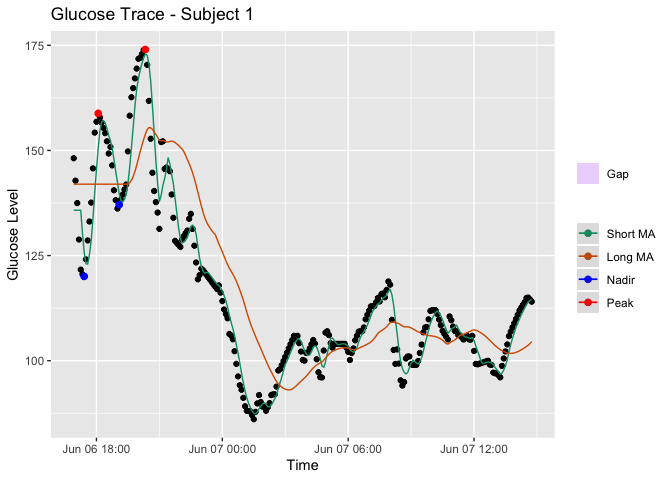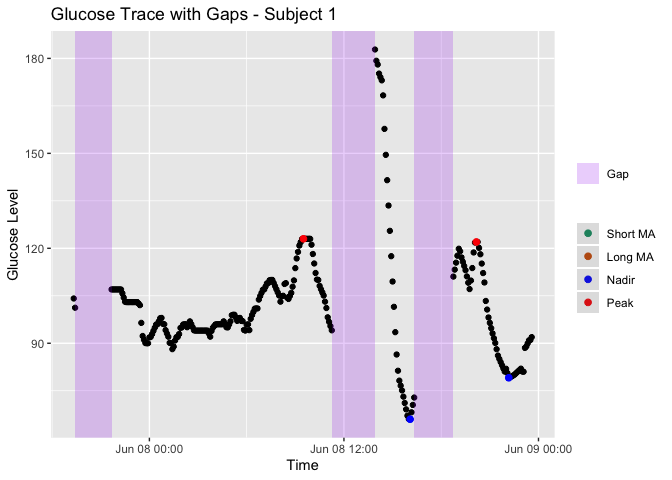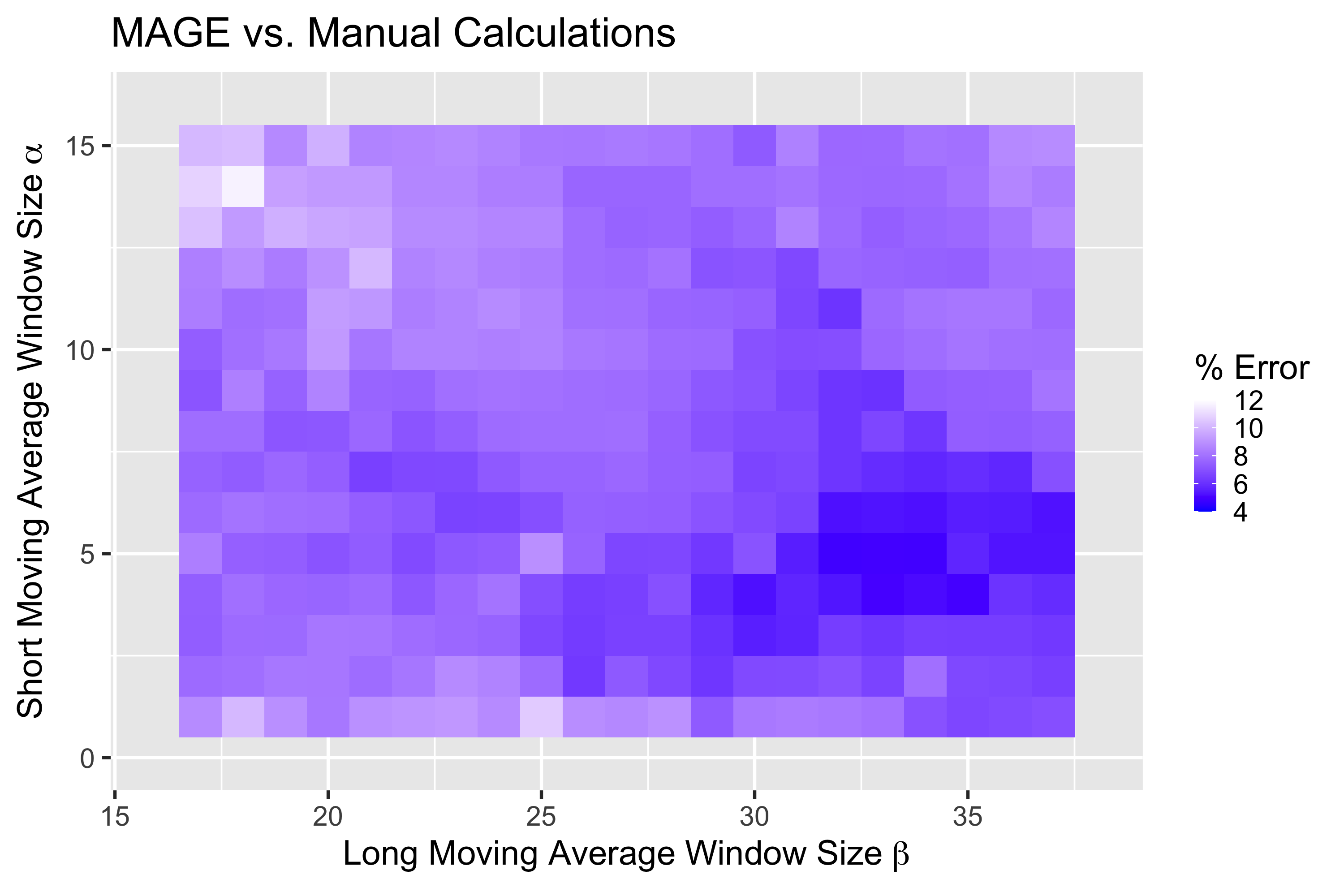# MAGE Algorithm

Mean Amplitude of Glycemic Excursions (MAGE) is a glucose variability metric that can be applied to CGM data. From iglu version 3 onward, the package includes two algorithms for MAGE calculation: (i) version = ‘ma’ uses short and long moving averages to computationally emulate the original definition, (ii) version = ‘naive’ is a naive approximation included for backwards compatibility with earlier versions of iglu. The moving average algorithm was published in the Journal of Diabetes Science and Technology: Open-source Algorithm to Calculate Mean Amplitude of Glycemic Excursions Using Short and Long Moving Averages, and the code to reproduce all tests can be found at the accompanying repository: Nathaniel-Fernandes/mage_algorithm_data. Below is a longer vignette describing the algorithm and the methods we used to test it. Some example code to help get started using MAGE in iglu is included in the Implementation section.

# Abstract

Background: Glucose variability is increasingly regarded as a risk factor for diabetes-related complications. The mean amplitude of glycemic excursions (MAGE) is a common measure of glucose variability. While its original definition requires manual calculations, there exist several software programs for automated calculations which have shown varying degrees of agreement. The objective of this study was to develop an automated algorithm for MAGE that would achieve high accuracy against the reference standard of manual calculations.

Methods: Manual calculations of MAGE were performed on 45 publicly available CGM profiles from a diverse set of patients (e.g., type 1 diabetes, type 2 diabetes, and those without diabetes). An automated algorithm for MAGE calculation was developed to identify peaks and nadirs of glycemic excursions based on the crosses of a short and long moving average of the glucose profile, and implemented in version 3 of R package iglu. The accuracy of the algorithm was evaluated relative to manual calculations using a five-fold cross-validation and compared to four other publicly available MAGE calculators.

Results: The newly developed algorithm had the median error of 1% relative to manual calculations. The median errors of the four other approaches including cgmanalysis, cgmquantify, EasyGV and algorithm in the earlier version of iglu were 20%, 78%, 11% and 42%, respectively.

Conclusions: The newly developed algorithm eliminates the need for tedious manual MAGE calculations and approximates the manual derivation better than existing approaches.

# Introduction

Glucose variability is increasingly regarded as a risk factor for diabetes-related complications beyond HbA1c [1, 2]. The mean amplitude of glycemic excursions (MAGE) is a commonly used measure of glucose variability. As defined by Service et al. , MAGE is the arithmetic mean of the amplitude (i.e. height) of glucose excursions that are greater than the standard deviation of the glucose values. MAGE is calculated manually by visually inspecting the glucose profiles. However, such manual approach to calculating MAGE is error-prone and impractical for large datasets of continuous glucose monitors (CGMs). Thus, an automated algorithm for MAGE calculation that approximates the manual reference is needed.

Several software programs for automated MAGE calculations have been developed, and these implementations can be divided into two categories: those that only return the numeric MAGE values, and those that additionally provide a visual display of the calculations . Examples in the first category include the Excel®-based workbook EasyGV , online GlyCulator2 , R package cgmanalysis , early versions of R package iglu , and Python package cgmquantify . Because these software programs do not output a visual display of the performed calculations, the user has no means to inspect the calculations and confirm accuracy. Examples in the second category include programs developed by Fritzsche  and Baghurst . However, the software developed by Fritzsche are not compatible with newer operating systems (e.g., Windows 10) to allow importing of CGM data. According to documentation for cgmanalysis  and cgmquantify , these packages provide free implementations of Baghurst’s algorithm, albeit they may not fully reflect the original implementation.

Comparison of GlyCulator, EasyGV, Fritzsche, and Baghurst algorithms demonstrates varying degrees of agreement across MAGE algorithms . While the aforementioned algorithms were compared to each other, they were not compared with the manual reference standard.

The objective of current study is to establish an approach of calculating MAGE that approximates well the results obtained manually. In developing an algorithm, the goal was to computationally emulate the manual method in Service et al.  based on the automated detection of peaks and nadirs tailored specifically towards CGM data, and to provide a freely available implementation with a visual display. To evaluate accuracy, the newly developed algorithm was compared with the manual derivations along with the other computational approaches.

# Methods

CGM Data and Manual MAGE Calculation

Publicly available CGM data were obtained from , from which 45 CGM traces were selected to represent a diverse set of patients (type 1 diabetes, type 2 diabetes and those without diabetes), see Table 1. Each CGM trace corresponds to one day of measurements (24 h), where a day is defined from midnight to midnight as it is customary to calculate MAGE daily. Manual MAGE calculations were performed on each of the 45 CGM traces following the original description of MAGE calculations by Service et al.  by two of the authors, who were unaware of the outcome of new derived automated analysis.

Dataset Diabetes Type Age group Subject ID Number of days used (CGM traces)
Hall et al. (2018) None Adults (> 18) 1636-69-001 5
1636-69-026 6
Tsalikian et al. (2005) Type 1 Children (10 - 18) 2 1
7 2
8 1
11 2
15 1
43 1
44 1
Dubosson et al. (2005) Type 1 Adults (> 18) 1 5
2 2
Broll et al. (2021) Type 2 Adults (> 18) Subject 1 1
Subject 2 5
Subject 3 1
Subject 4 5
Subject 5 6

Table 1: Summary of the 45 CGM traces used for manual calculations. The first three datasets have been obtained as described in . The last dataset is available within R package iglu .

The Algorithm

The original MAGE description in Service et al.  requires (i) identification of turning points (e.g., peaks and nadirs) for calculation of glucose excursions; (ii) calculation of the mean of excursion amplitudes (i.e., heights) by filtering only those that are above the threshold of one standard deviation. To the best of our knowledge, our implementations for both parts are unique.

1. Identification of peaks and nadirs. The proposed algorithm uses the crosses of short and long moving averages to identify time intervals where a peak or nadir may exist. Let $$\alpha$$ be the window size for the short moving average, and 𝛽 be the window size for the long moving average, where $$\alpha < \beta$$. A peak or nadir must exist on the intervals that are bounded by the crosses of the two moving averages as demonstrated in Figure 1. This occurs because the shorter moving average is more affected by the local variation in the glucose values than the longer moving average. The glucose value at a peak or nadir (i.e., $$p_i$$) is then determined as the maximum or minimum glucose value, respectively, between the two crossing points. By default, the first and last glucose values are always treated as turning points. A distinct advantage of using moving averages to identify the turning points is that they inherently smooth out local fluctuations and noise within larger trends in a glucose profile. The amount of smoothing can be tuned by varying window size parameters $$\alpha$$ and $$\beta$$. By default, the algorithm uses $$\alpha = 5$$ and $$\beta = 32$$ as these values lead to high accuracy when compared to the manual MAGE calculations.

2. Eliminating Excursions and Calculation of Mean Amplitudes. Given the glucose values $$p_i$$ at the turning points (either peaks or nadirs), the amplitude of an excursion is calculated as |$$p_{i + 1}-p_i$$|. The amplitude value corresponds to the ascending segment if $$p_i$$ corresponds to a nadir, and to the descending segment if $$p_i$$ corresponds to a peak. First, we check whether the amplitudes exceed one standard deviation threshold. If an amplitude value is below the threshold, the algorithm will skip the corresponding turning points, and recalculate the amplitudes based on the remaining $$p_i$$ (this allows to absorb a smaller excursion within a larger excursion). Secondly, to prevent double counting excursions, only ascending (MAGE+) or descending (MAGE–) excursion amplitudes are averaged. When excursions are symmetric, MAGE+ = MAGE–, however in general they may disagree . By default, the algorithm follows the description in Service et al. , and calculates MAGE+ or MAGE– depending on which type of excursion first crosses the one SD threshold.

Implementation
The newly developed algorithm for MAGE calculation can be found from version 3 onward in R package iglu  as well as the accompanying GUI via Shiny App  - both are free and open-source. Implementation in a script-based programming language like R allows the creation of reproducible scripts for all data processing and MAGE calculation steps, and the availability of a point-and-click GUI meets the needs of researchers with limited programming experience. By default, the algorithm uses $$\alpha = 5$$ and $$\beta = 32$$, however these choices can be adjusted by the user.

# load package and example data
library(iglu)
# mage calculation using default parameters
mage(example_data_5_subject)
#> # A tibble: 5 × 2
#> # Rowwise:
#>   id         MAGE
#>   <fct>     <dbl>
#> 1 Subject 1  87.2
#> 2 Subject 2 111.
#> 3 Subject 3 115.
#> 4 Subject 4  70.1
#> 5 Subject 5 146.
# Adjust default window sizes in mage calculation
mage(example_data_5_subject, short_ma = 3, long_ma = 30)
#> # A tibble: 5 × 2
#> # Rowwise:
#>   id         MAGE
#>   <fct>     <dbl>
#> 1 Subject 1  91.8
#> 2 Subject 2 110.
#> 3 Subject 3 114.
#> 4 Subject 4  70.3
#> 5 Subject 5 146.

In addition to providing a numerical MAGE value, the software allows the creation of glucose trace plots that highlight the selected peaks and nadirs used for MAGE calculation.

# subset data to better see identified peaks and nadirs
fig1data <- example_data_1_subject[1:200, ]
# plot using mage function with plot = TRUE and show_ma = TRUE
mage(fig1data, plot = TRUE, show_ma = TRUE, title = "Glucose Trace - Subject 1")Figure 1: Glucose trace of Subject 2 with Type 2 diabetes from Broll et al. , the solid green line is a short MA with $$\alpha$$ = 5 and the solid orange line is a long MA with $$\beta$$ = 32. The peaks and nadirs occur between the crosses of short and long moving averages. By default, the algorithm calculates MAGE+ as in this profile the first segment to exceed one SD threshold is ascending. The provided graphical display in iglu software  automatically highlights only those peaks (red) and nadirs (blue) that are used for final calculation.

Additionally, the plot will highlight any gaps in the data due to missing readings.

# subset data to better show gaps
fig2data <- example_data_1_subject[200:400, ]
# plot using mage function with plot = TRUE
mage(fig2data, plot = TRUE, title = "Glucose Trace with Gaps - Subject 1")Figure 2. Glucose trace of Subject 5 with Type 2 diabetes from Broll et al.  with regions of missing glucose readings automatically highlighted using iglu software .

Assessment of Accuracy
The accuracy of the proposed algorithm was evaluated against 45 manual calculations. For each CGM trace, the accuracy was measured as the relative percent difference in the automatically calculated MAGE value and the manual MAGE value, that is: |MAGEalgorithm - MAGEmanual|/MAGEmanual⋅ 100%.

To find optimal combination of window sizes, we considered $$\alpha \in [1, 15]$$ and $$\beta \in [16, 38]$$, and recorded the mean errors across 45 CGM traces for each pair of ($$\alpha, \beta$$) values. The optimal ($$\alpha, \beta$$) pair was determined as the one that leads to the minimal in-sample error. Since the obtained minimal error is a biased assessment of accuracy as the optimal ($$\alpha, \beta$$) were selected based on the same 45 traces, unbiased assessment of accuracy using five-fold cross-validation was also performed. The 45 CGM traces were randomly split into five groups of nine samples each. The best ($$\alpha, \beta$$) combination was then chosen using the four out of five groups, and used to evaluate the accuracy on the remaining group. The best combination was defined as the one that gave the lowest percent error compared to manual calculations. This out-of-sample evaluation was performed on all five groups.

The accuracy of EasyGV , cgmanalysis , cgmquantify , and the MAGE algorithm implemented in the earlier version of iglu  was also determined against the manual reference derivation. The latter is a naive simplification of original MAGE definition, as it calculates MAGE as the mean of absolute differences between glucose values and the glucose mean that are larger than one standard deviations (and thus does not attempt to explicitly identify peaks and nadirs). We use iglu_ma to denote the proposed algorithm based on moving averages, and iglu_naive to denote the naive algorithm used in earlier versions of the software. We were unable to evaluate GlyCulator2  because the software requires CGM trace to have at least 576 time points, and thus returns NA when applied to 24-hour CGM data. We were also unable to evaluate Fritzsche  as importing the CGM data into the provided user interface on Windows 10 lead to errors.

# Results

The optimal choice of window sizes ($$\alpha, \beta$$) for the proposed algorithm was determined to be (5, 32) as this combination minimized the average error relative to 45 manual calculations. Figure 3 shows a heatmap of the percent average error (across 45 traces) as a function of $$\alpha$$ and $$\beta$$, where the darkest regions represent the most favorable combinations of $$\alpha$$ and $$\beta$$. The minimum corresponds to the average error of 4.8%. The corresponding median relative error was much smaller, 1.1%, with the inter-quartile range of [0.3%, 3.6%]. As expected, the out-of-sample cross-validated errors were larger, but still relatively low. The average cross-validated error was 5.8%, with the median of 1.3% and inter-quartile range of [0.3%, 6.6%].Figure 3. Heatmap of average errors over 45 CGM traces of automatically calculated MAGE values via proposed algorithm relative to manual MAGE values. The short moving average window size $$\alpha \in [1, 15]$$ and the long moving average window size $$\beta \in [16, 38]$$. The darker regions correspond to smaller errors, with the minimal error of 4.8% achieved at $$\alpha = 5$$, $$\beta = 32$$.

Compared to the other available automated algorithms, the next best results were achieved by EasyGV, which had an average error of 23.4%, the median error of 11.3% and the IQR of [6.0%, 22.3%]. In constrast, cgmanalysis, iglu_naive, and cgmquantify all had significantly higher errors, with median errors (and interquartile range) of 20.3% [8.6%, 32.1%], 42.0% [32.6%, 48.3%], and 78.4% [30.8%, 131.9%], respectively. Figure 4 shows boxplots of relative errors of all algorithms on 45 CGM traces.